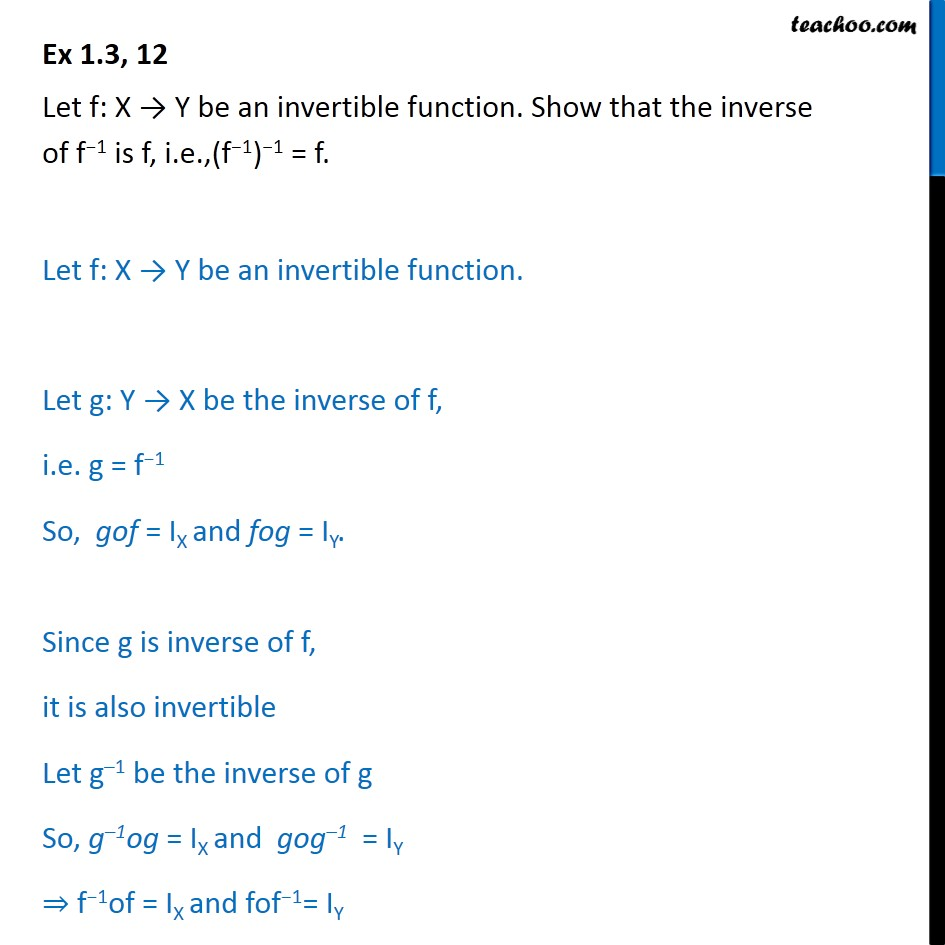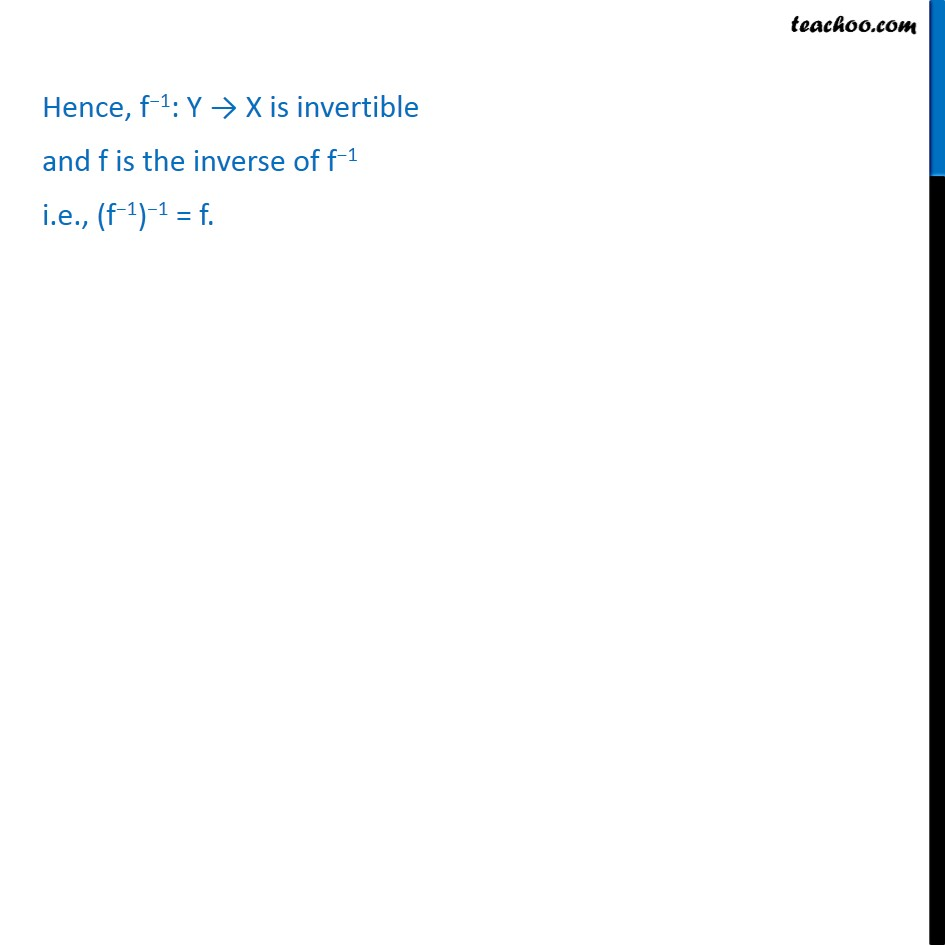Inverse of a function

Chapter 1 Class 12 Relation and Functions
Serial order wiseLearn in your speed, with individual attention - Teachoo Maths 1-on-1 Class

### Transcript

Ex 1.3, 12 Let f: X Y be an invertible function. Show that the inverse of f 1 is f, i.e.,(f 1) 1 = f. Let f: X Y be an invertible function. Let g: Y X be the inverse of f, i.e. g = f 1 So, gof = IX and fog = IY. Since g is inverse of f, it is also invertible Let g 1 be the inverse of g So, g 1og = IX and gog 1 = IY f 1of = IX and fof 1= IY Hence, f 1: Y X is invertible and f is the inverse of f 1 i.e., (f 1) 1 = f.